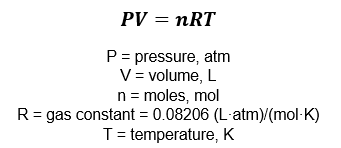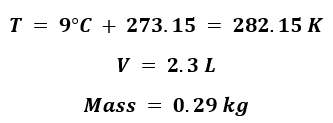# Problem: A scuba divers tank contains 0.29 kg of O2 compressed into a volume of 2.3 L.Calculate the gas pressure inside the tank at 9 oC.

###### FREE Expert Solution

Use the ideal gas equation to solve for pressure:Given: Make sure to convert to the necessary units requiredFind the number of moles of the O2(g):

92% (441 ratings)###### Problem Details

A scuba divers tank contains 0.29 kg of O2 compressed into a volume of 2.3 L.

Calculate the gas pressure inside the tank at 9 oC.